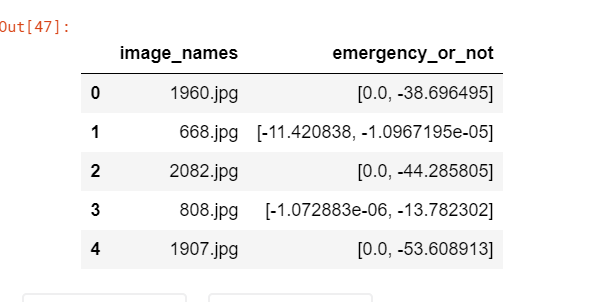# Binary Image classification using Pytorch

Hi All,

I am doing a binary image classification using pytorch and I am using CNN architecture to do that.

In the final layer of architecture - do I need to give number of outputs parameter as “2” or “1”? I placed as 1 and My final target columns have values [0,1,0,0,0,…]

Here is my code for the final layer:

``````        nn.Linear(128, 64),
nn.ReLU(),
nn.Linear(64, 1),
nn.Sigmoid()
)
``````

Please clarify if it is correct. I am having some follow up questions on this.

–Shravan

For binary classification, the number of units in the last fully-connected layer can be 1 or 2.

• If you use Sigmoid activation, you must apply 1 unit for the las FC layer (Your above code is correct). In this case, the loss function should be BCE.
• If you use Softmax activation, you should apply 2 units. In this case, the loss function should be CE.
1 Like

I am getting -ve value predictions for the binary classification problem. Any idea where is the mistake? my train and validation is working fine and showing a decent 75% accuracy.Here is the prediction code:

how to get the output in the format of 1/0?